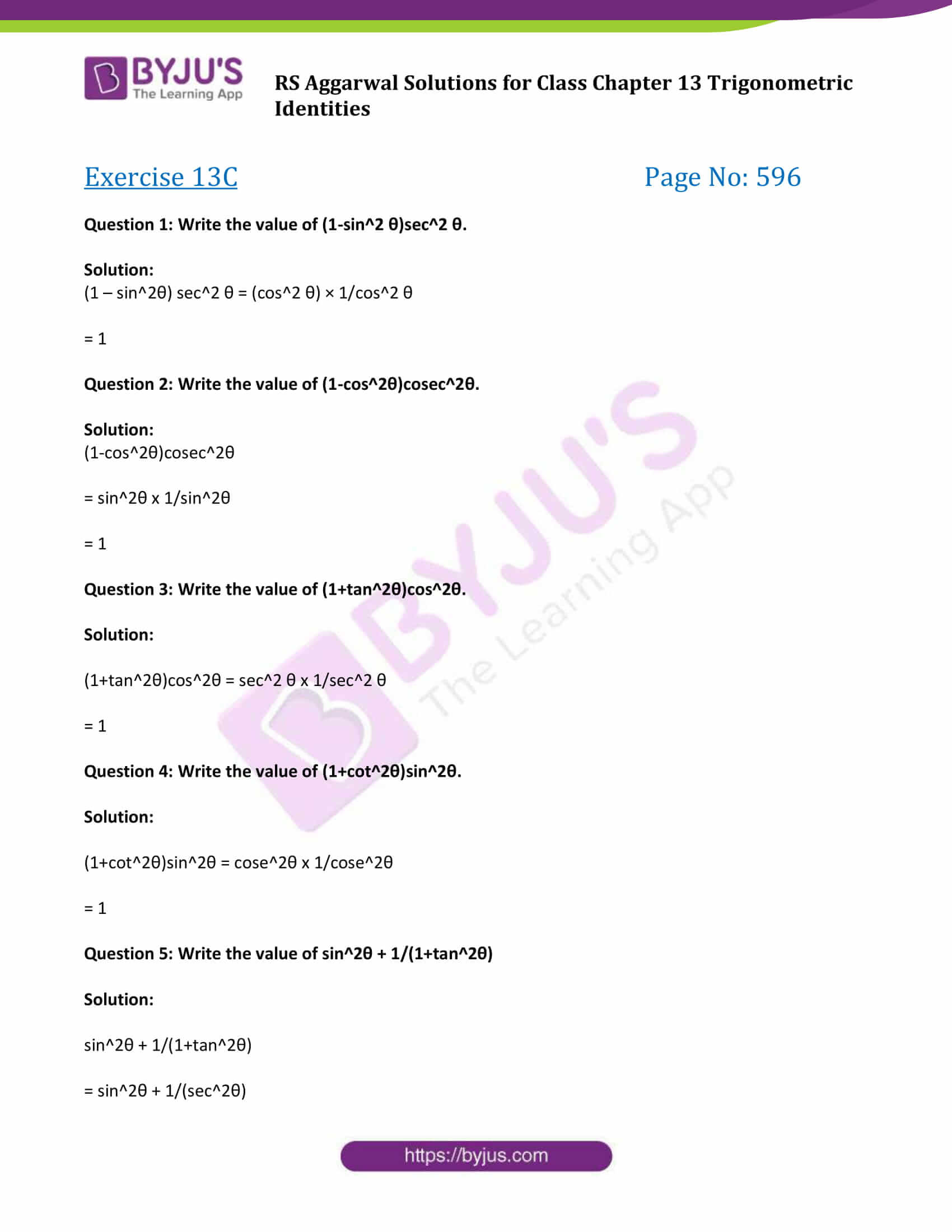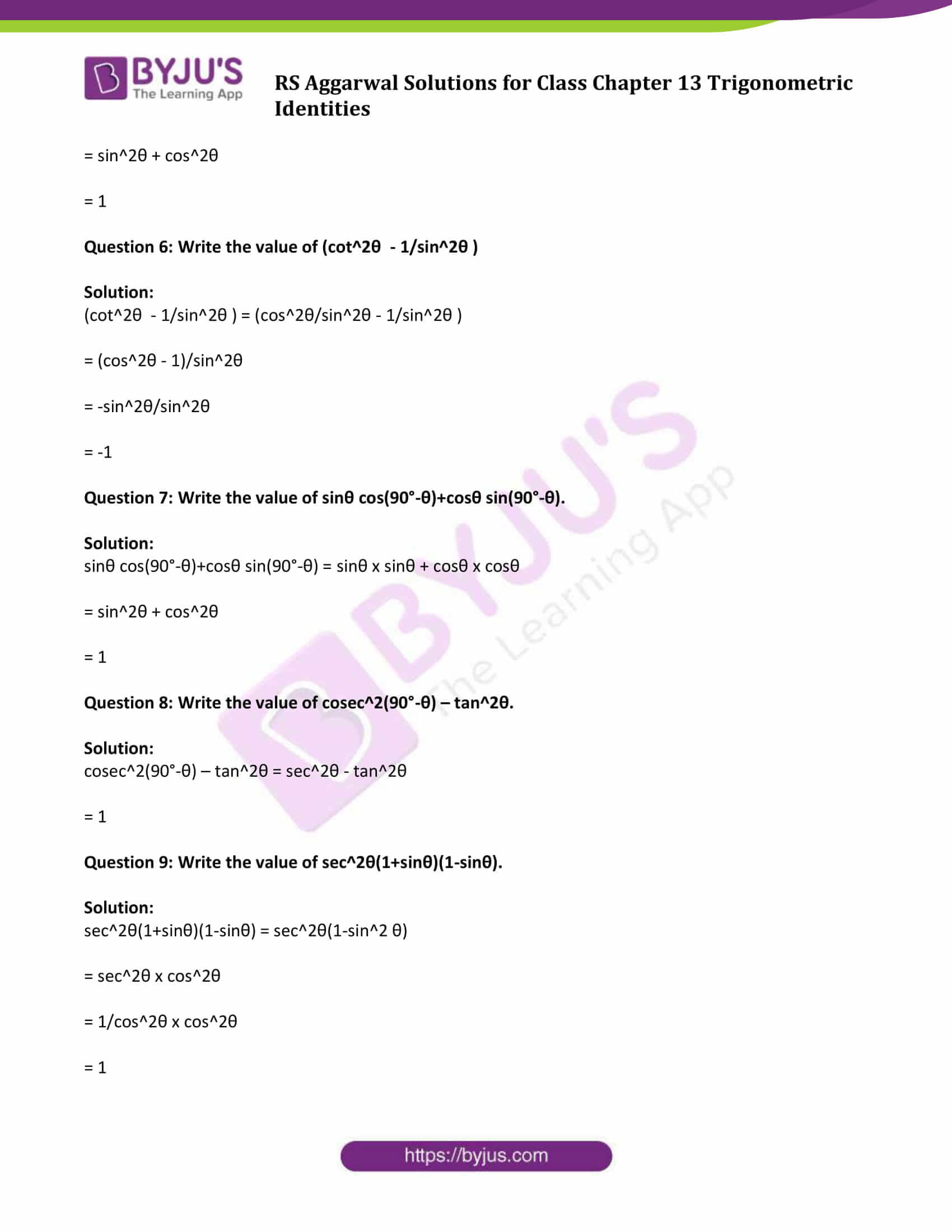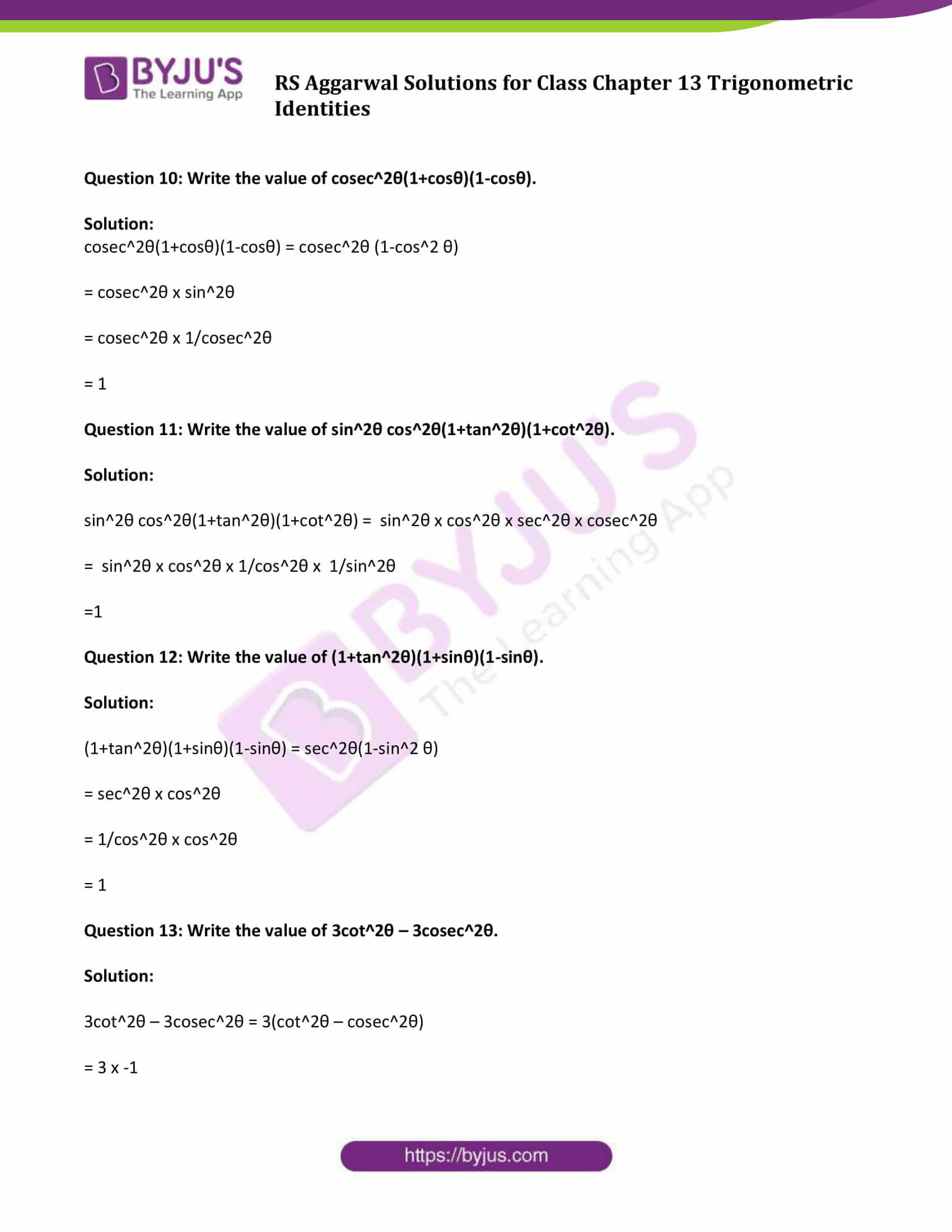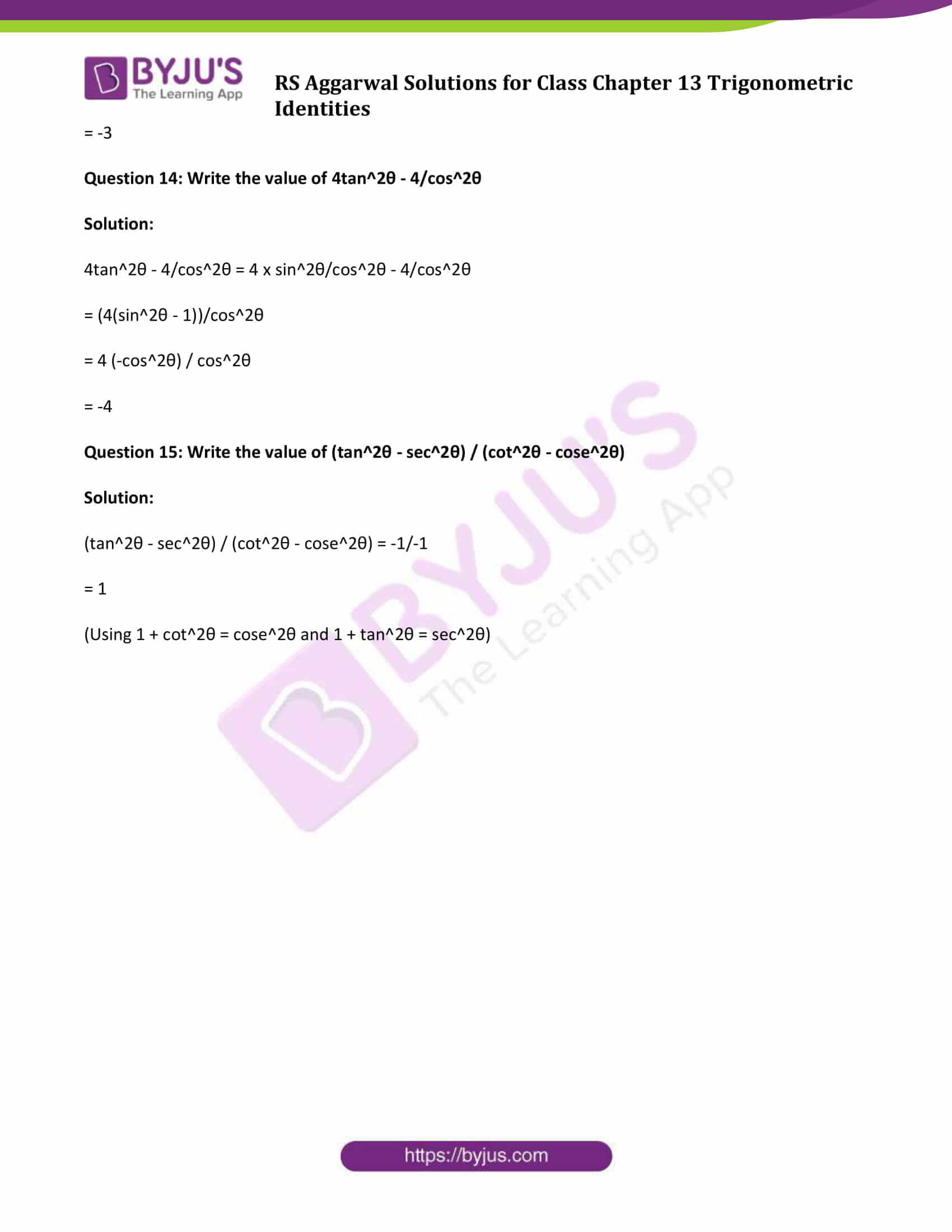# R S Aggarwal Solutions for Class 10 Maths Chapter 13 Trigonometric Identities Exercise 13C

R S Aggarwal solutions Class 10 Maths is a helpful resource prepared by subject experts to help students in understanding the various concepts covered in class 10 syllabus. Chapter 13- Trigonometric identities is one of the key chapters and consists of important topics that are often asked in class 10 Maths examination. Practising these questions along with solutions will affect the brainstorming capability among students.

## Download PDF of R S Aggarwal Solutions for Class 10 Maths Chapter 13 Trigonometric Identities Exercise 13C### Access other exercise solutions of Class 10 Maths Chapter 13 Trigonometric Identities

Exercise 13 A Solutions

Exercise 13 B Solutions

### Access Answers to Maths R S Aggarwal Class 10 Chapter 13 – Trigonometric Identities Exercise 13C

Question 1: Write the value of (1-sin2 θ)sec2 θ.

Solution:

(1 – sin2θ) sec2 θ = (cos2 θ) × 1/cos2 θ

= 1

Question 2: Write the value of (1-cos2θ)cosec2θ.

Solution:

(1-cos2θ)cosec2θ

= sin2θ x 1/sin2θ

= 1

Question 3: Write the value of (1+tan2θ)cos2θ.

Solution:

(1+tan2θ)cos2θ = sec2 θ x 1/sec2 θ

= 1

Question 4: Write the value of (1+cot2θ)sin2θ.

Solution:

(1+cot2θ)sin2θ = cose2θ x 1/cose2θ

= 1

Question 5: Write the value of sin2θ + 1/(1+tan2θ)

Solution:

sin2θ + 1/(1+tan2θ)

= sin2θ + 1/(sec2θ)

= sin2θ + cos2θ

= 1

Question 6: Write the value of (cot2θ – 1/sin2θ )

Solution:

(cot2θ – 1/sin2θ ) = (cos2θ/sin2θ – 1/sin2θ )

= (cos2θ – 1)/sin2θ

= -sin2θ/sin2θ

= -1

Question 7: Write the value of sinθ cos(90°-θ)+cosθ sin(90°-θ).

Solution:

sinθ cos(90°-θ)+cosθ sin(90°-θ) = sinθ x sinθ + cosθ x cosθ

= sin2θ + cos2θ

= 1

Question 8: Write the value of cosec2(90°-θ) – tan2θ.

Solution:

cosec2(90°-θ) – tan2θ = sec2θ – tan2θ

= 1

Question 9: Write the value of sec2θ(1+sinθ)(1-sinθ).

Solution:

sec2θ(1+sinθ)(1-sinθ) = sec2θ(1-sin2 θ)

= sec2θ x cos2θ

= 1/cos2θ x cos2θ

= 1

Question 10: Write the value of cosec2θ(1+cosθ)(1-cosθ).

Solution:

cosec2θ(1+cosθ)(1-cosθ) = cosec2θ (1-cos2 θ)

= cosec2θ x sin2θ

= cosec2θ x 1/cosec2θ

= 1

Question 11: Write the value of sin2θ cos2θ(1+tan2θ)(1+cot2θ).

Solution:

sin2θ cos2θ(1+tan2θ)(1+cot2θ) = sin2θ x cos2θ x sec2θ x cosec2θ

= sin2θ x cos2θ x 1/cos2θ x 1/sin2θ

=1

Question 12: Write the value of (1+tan2θ)(1+sinθ)(1-sinθ).

Solution:

(1+tan2θ)(1+sinθ)(1-sinθ) = sec2θ(1-sin2 θ)

= sec2θ x cos2θ

= 1/cos2θ x cos2θ

= 1

Question 13: Write the value of 3cot2θ – 3cosec2θ.

Solution:

3cot2θ – 3cosec2θ = 3(cot2θ – cosec2θ)

= 3 x -1

= -3

Question 14: Write the value of 4tan2θ – 4/cos2θ

Solution:

4tan2θ – 4/cos2θ = 4 x sin2θ/cos2θ – 4/cos2θ

= (4(sin2θ – 1))/cos2θ

= 4 (-cos2θ) / cos2θ

= -4

Question 15: Write the value of (tan2θ – sec2θ) / (cot2θ – cose2θ)

Solution:

(tan2θ – sec2θ) / (cot2θ – cose2θ) = -1/-1

= 1

(Using 1 + cot2θ = cose2θ and 1 + tan2θ = sec2θ)

## R S Aggarwal Solutions for Class 10 Maths Chapter 13 Trigonometric Identities Exercise 13C

Class 10 Maths Chapter 13 Trigonometric Identities Exercise 13C is based on the Pythagorean formula for tangents and secants and identities expressing trig functions in terms of their supplements. Download R S Aggarwal Solutions Class 10 Chapter 13 and score well in your exams.